# Boylestad: MCQ in Power Supplies (Voltage Regulators)

(Last Updated On: December 2, 2019)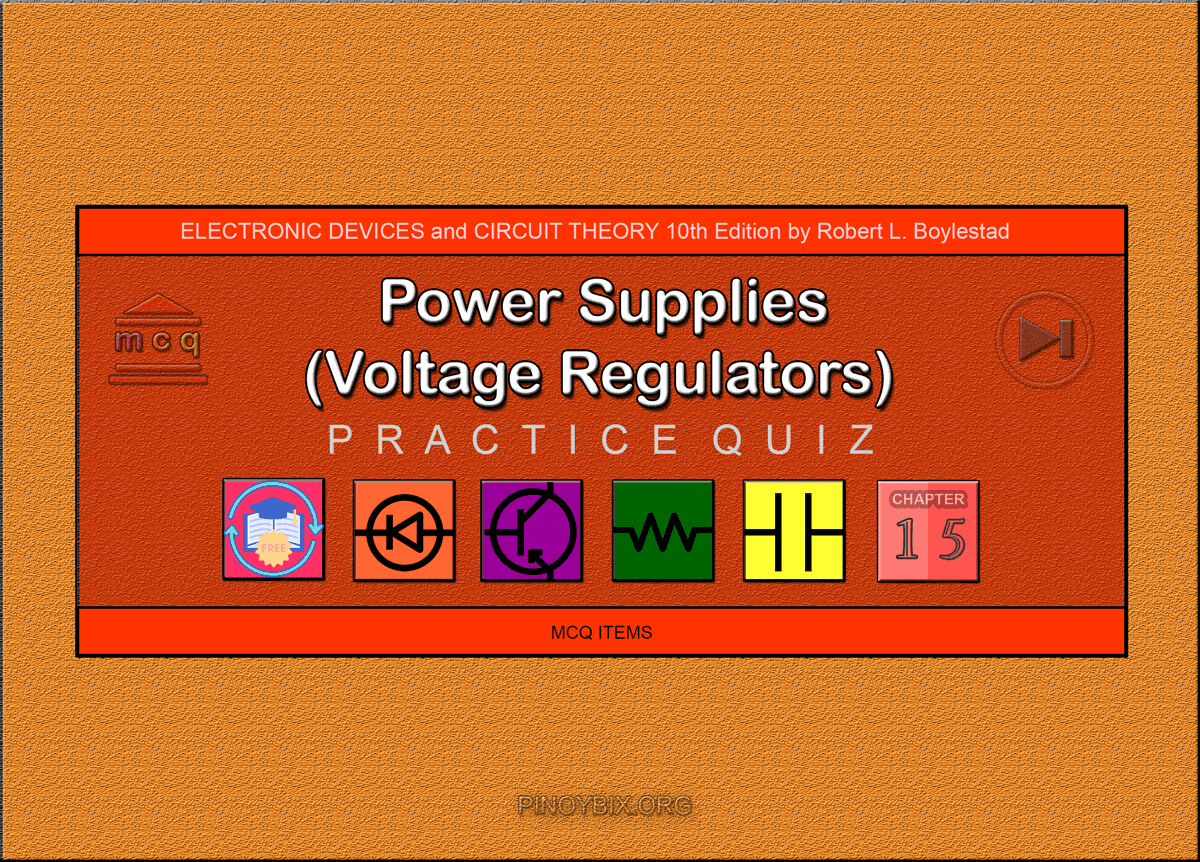This is the Multiple Choice Questions in Power Supplies (Voltage Regulators) from the book Electronic Devices and Circuit Theory 10th Edition by Robert L. Boylestad. If you are looking for a reviewer in Electronics Engineering this will definitely help. I can assure you that this will be a great help in reviewing the book in preparation for your Board Exam. Make sure to familiarize each and every questions to increase the chance of passing the ECE Board Exam.

#### Online Questions and Answers Topic Outline

• MCQ in Introduction to Power Supplies | MCQ in General Filter Considerations | MCQ in Capacitor Filter | MCQ in RC Filter | MCQ in Discrete Transistor Voltage Regulation | MCQ in IC Voltage Regulators

#### Practice Exam Test Questions

Choose the letter of the best answer in each questions.

1. In which of the following applications is a pulsating dc voltage suitable?

A. Battery charger

C. Stereo system

D. Computer

Solution:

2. Calculate the ripple of a filter output having a 20-V dc component and a 1.7 Vr(rms) ac component.

A. 6%

B. 8.5%

C. 85%

D. 58%

Solution:

3. Calculate the voltage regulation of a power supply having VNL = 50 V and VFL = 48 V.

A. 4.17%

B. 5.2%

C. 6.2%

D. 7.1%

Solution:

4. Across which of the following components of a power supply does the average (dc) voltage exist?

A. Diodes

B. Secondary of the transformer

C. Capacitor filter

D. None of the above

Solution:

5. Calculate the ripple voltage of a full-wave rectifier with a 75-µF filter capacitor connected to a load drawing 40 mA.

A. 1.20 V

B. 1.28 V

C. 1.32 V

D. 1.41 V

Solution:

6. What is the ratio of the period of the output voltage to the period of the input voltage in a full-wave rectifier?

A. 0

B. 0.5

C. 1

D. 2

Solution:

7. A ______ -wave rectified signal has less ripple than a ______ -wave rectified signal and is thus better to apply to a filter.

A. full, half

B. half, full

Solution:

8. If the value of full-load voltage is the same as the no-load voltage, the voltage regulation calculated is _____ %, which is the best expected.

A. 0

B. 1

C. 99

D. 100

Solution:

9. In which period is the capacitor filter charged in a full-wave rectifier?

A. The time during the positive cycle

B. The time during which the diodes are not conducting

C. The time during which the diode(s) is (are) conducting

D. The time during the negative cycle

Solution:

10. In which period is the capacitor filter discharged through the load in a full-wave rectifier?

A. The time during the positive cycle

B. The time during which the diodes are not conducting

C. The time during which the diode(s) is (are) conducting

D. The time during the negative cycle

Solution:

11. If a peak rectified voltage for the full-wave filter circuit is 40 V, calculate the filter dc voltage if C = 75 µF and load current is 40 mA.

A. 27.9 V

B. 32.12 V

C. 37.78 V

D. 40 V

Solution:

12. Calculate the ripple of a capacitor filter for a peak rectified voltage of 40 V, a capacitor value C = 75 µF, and a load current of 40 mA.

A. 3.2%

B. 3.59%

C. 4.03%

D. 4.59%

Solution:

13. What is the ratio of the peak ripple voltage level to its rms voltage level?

A. √3

B. √2

C. √3/2

D. √2/2

Solution:

14. The larger the value of the capacitor, the smaller the peak current drawn through the rectifying diodes.

A. True

B. False

Solution:

15. What is the purpose of an additional RC filter section in a power supply circuit?

A. Increase the dc voltage component

B. Increase the ac voltage component

C. Decrease the ac voltage component

D. None of the above

Solution:

16. Calculate the dc voltage across a 2-kΩ load for an RC filter section (R = 50 Ω, C = 20 µF). The dc voltage across the initial filter capacitor is Vdc = 50 V.

A. 40.78 V

B. 42.78 V

C. 45.78 V

D. 48.78 V

Solution:

17. The purpose of the added RC section is to pass most of the dc component while reducing as much of the ac component as possible.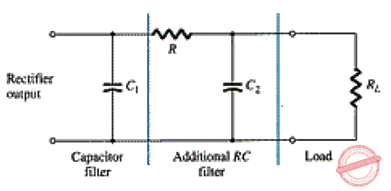A. True

B. False

Solution:

18. This circuit is an example of the ac equivalent of an RC filter.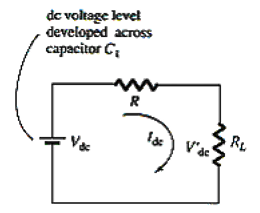A. True

B. False

Solution:

19. For a full-wave rectifier with ac ripple at 120 Hz, the impedance of a capacitor can be calculated using XC = ______.

A. 0.707 ÷ C

B. 1.414 ÷ C

C. 1.3 ÷ C

D. 0.785 ÷ C

Solution:

20. In a simple series regulator circuit, which of the following components is the controlling element?

B. Zener diode

C. Transistor Q1

D. None of the above

Solution:

21. In this improved series regulator circuit, which of the following components is the sampling circuit?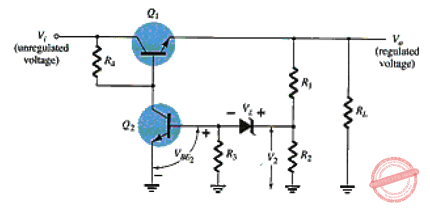A. Zener diode

C. Either of the two transistors Q1 or Q2

D. Resistors R1 and R2

Solution:

22. In this op-amp series regulator circuit, which of the following components is the comparator circuit?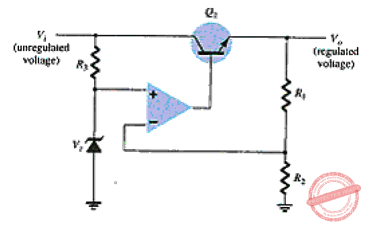A. Op-amp

B. Transistor Q1

C. R1 and R2 resistors

D. Zener diode

Solution:

23. What regulated output voltage is provided for the following circuit elements: R1 = 15 kΩ, R2 = 35 kΩ, and VZ = 11.2 V?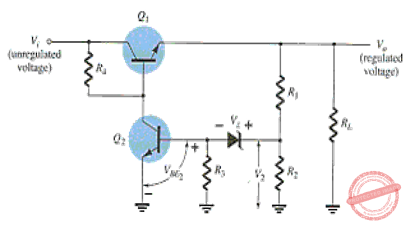A. 16.50 V

B. 17 V

C. 17.35 V

D. 18.25 V

Solution:

24. Which component(s) set(s) the voltage across the load in a basic transistor shunt regulator?

A. Zener diode

B. Transistor base-emitter voltage

C. Both the Zener diode and the transistor base-emitter voltage

D. None of the above

Solution:

25. In an improved shunt regulator, which of the following components sets the reference voltage?

A. Transistor Q1

B. Zener diode

C. Transistor Q2

D. RS

Solution:

26. For what range of load current can voltage regulators be selected for operation?

A. Hundreds of picoamperes to tens of nanoamperes

B. Hundreds of picoamperes to tens of milliamperes

C. Hundreds of milliamperes to tens of amperes

D. None of the above

Solution:

27. For what range of fixed regulated voltages do the series 78xx regulators provide regulation?

A. –5 V to +24 V

B. +5 V to +24 V

C. –5 V to –24 V

D. None of the above

Solution:

28. What is the typical dropout voltage for the 7812 fixed positive voltage regulator?

A. 4 mV

B. 100 mV

C. 1.5 V

D. 2 V

Solution:

29. How many diodes conduct in the full-wave bridge rectifier while the capacitor is being charged?

A. 1

B. 2

C. 3

D. 4

Solution:

30. What is the range of the voltage level of the LM317 adjusted voltage regulator?

A. 0 V to 5 V

B. 1.2 V to 37 V

C. –5 V to –24 V

D. 5 V to 24 V

Solution:

31. What are the typical values of Vref and Iadj for the LM317 adjustable voltage regulator?

A. 1.0 V, 100 mA

B. 1.5 V, 100 mA

C. 1.25 V, 100 μA

D. 1.25 V, 10 mA

Solution:

32. The 7812 regulator IC provides _____.

A. 5 V

B. –5 V

C. 12 V

D. –12 V

Solution:

33. The 7912 regulator IC provides _____ .

A. 5 V

B. –5 V

C. 12 V

D. –12 V

Solution:

34. The 7905 regulator IC provides _____.

A. 5 V

B. –5 V

C. 12 V

D. –12 V

Solution:

35. The 7805 regulator IC provides _____.

A. 5 V

B. –5 V

C. 12 V

D. –12 V

Solution:

#### FILL-IN-THE-BLANKS

1. A complete power supply has a _____.

A. rectifier

B. filter

C. voltage regulator

D. All of the above

Solution:

2. The output resulting from a rectifier is a(n) ______.

A. ac voltage

B. pure dc voltage

C. pulsating dc voltage

D. None of the above

Solution:

3. The ______ the ac variation with respect to the dc level, the ______ the filter circuit’s operation.

A. smaller, better

B. larger, better

C. smaller, worse

D. None of the above

Solution:

4. A dc voltmeter reads the _______ while measuring a pulsating dc voltage.

A. ac component of the signal

B. average of the signal

C. peak of the pulsating signal

D. None of the above

Solution:

5. The filter output voltage of a power supply _______ when load current is drawn from the supply.

A. remains the same

B. is increased

C. is reduced

D. None of the above

Solution:

6. The ______ the voltage regulation, the ______ the operation of the voltage supply circuit.

A. smaller, better

B. larger, better

C. smaller, worse

D. None of the above

Solution:

7. A full-wave rectified signal has ______ dc component and ______ ripple than (as) the half-wave rectified voltage.

A. a larger, more

B. a smaller, less

C. the same, less

D. a larger, less

Solution:

8. If the value of the full-load voltage is the same as the no-load voltage, the voltage regulation calculated is _______.

A. 0%

B. a negative percentage

C. a positive percentage

D. None of the above

Solution:

9. In a half-wave rectifier, the dc voltage level is ______ the ripple voltage level.

A. smaller than

B. the same as

C. larger than

D. None of the above

Solution:

10. In a full-wave rectifier, the dc voltage level is ______ the ripple voltage level.

A. smaller than

B. the same as

C. larger than

D. None of the above

Solution:

11. In a full-wave rectifier, if no load were connected across the capacitor, the output voltage would ideally be a(n) ______.

A. ac voltage

B. constant dc voltage

C. pulsating dc voltage

D. ramp voltage

Solution:

12. The output of a loaded power supply is ______ that of the unloaded.

A. the same as

B. larger than

C. smaller than

D. None of the above

Solution:

13. The charging and discharging of the capacitor filter take ______ of the period of the input voltage.

A. 0.25

B. 0.5

C. 0.75

D. 1

Solution:

14. The frequency of the output voltage of a full-wave rectifier is ______ the frequency of its input voltage.

A. the same as

B. twice

C. one-half

D. one-third

Solution:

15. The ripple voltage Vr is a result of the ______.

A. conduction of the diode(s)

B. transformer windings

C. charging and discharging of the capacitor

Solution:

16. The ______ values of capacitor filter provide ______ ripple and ______ average voltage.

A. larger, more, higher

B. smaller, less, lower

C. smaller, more, higher

D. larger, less, higher

Solution:

17. The ______ the diode conduction time, the ______ the amount of the charging current through the capacitor filter.

A. shorter, larger

B. shorter, smaller

C. longer, larger

D. None of the above

Solution:

18. In a current-limiting circuit, ______ provide(s) the limiting of the maximum load current.

A. the Zener diode

B. the short-circuit resistor Rsc

C. the transistor Q1

D. both the short-circuit resistor Rsc and transistor Q2

Solution:

19. In a foldback configuration, limiting the current reduces ______, protecting the load from overcurrent as well as protecting the regulator.

A. the output voltage

B. the output current

C. both the output voltage and output current

D. None of the above

Solution:

20. A type of regulator circuit that is quite popular for its efficient transfer of power to the load is the ______.

A. current-limiting voltage regulator

B. switching regulator

C. foldback limiting regulator

D. op-amp series regulator

Solution:

21. Regulator IC units contain the circuitry for the _______.

A. reference source

B. comparator amplifier

C. control device and overload protection

D. All of the above

Solution:

22. IC units provide regulation of _______.

A. a fixed positive voltage

B. a fixed negative voltage

D. All of the above

Solution:

23. The specification sheet for the 7812 fixed positive voltage regulator shows that the output voltage could be as low as ______ or as high as ______.

A. 11.5 V, 12.5 V

B. 11.2 V, 12.2 V

C. 11 V, 13 V

D. 8 V, 15 V

Solution:

24. The series 7900 ICs are _______.

A. positive voltage regulators

B. negative voltage regulators

C. both positive and negative voltage regulators

Solution:

25. The _______ is (are) an adjustable voltage regulator.

A. series 7800 ICs

B. series 7900 ICs

C. LM317

D. None of the above

Solution:

### Complete List of Chapter Quiz in Electronic Devices and Circuit Theory

P inoyBIX educates thousands of reviewers and students a day in preparation for their board examinations. Also provides professionals with materials for their lectures and practice exams. Help me go forward with the same spirit.

“Will you subscribe today via YOUTUBE?”

Subscribe

PinoyBIX Engineering. © 2014-2020 All Rights Reserved | How to Donate? |